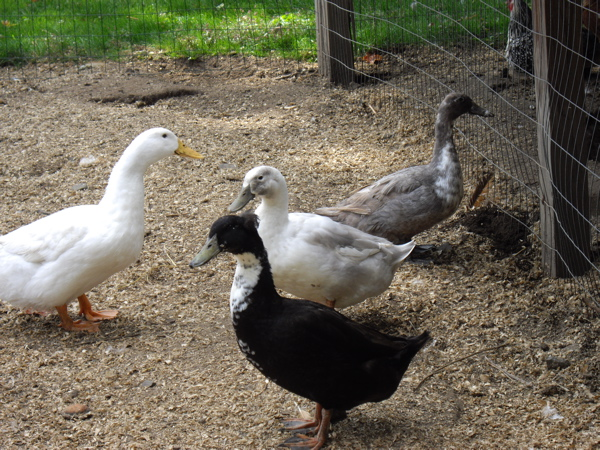### Discrete Mathematics with Ducks

###### by dr. sarah-marie belcastroThere is a one-to-one correspondence between the ducks on the left and the eggs on the right. Actually, there are many one-to-one correspondences... How many?Discrete Mathematics with Ducks (second edition CRC Press 2018; first edition AK Peters/CRC Press 2012) is a textbook intended for a sophomore-level course in discrete mathematics. It does not have any mathematical prerequisites and it does not assume any prior exposure to proofwriting. Still, the focus is firmly on actual discrete mathematics content rather than general mathematical background. Discrete Mathematics with Ducks is particularly amenable to use by faculty who like a discovery-based approach; in-class activities are supplied for every topic, and about half of the topics are presented so that students' first exposure to the material is via explorations in class. For this reason, the book is also amenable to self-study. However, the mathematical presentation would also work with more common teaching styles. There are lots and lots of homework problems as well, including very straightforward problems at the end of explanatory sections, the usual sorts of homework problems at the end of each chapter, and 50 additional problems at the end of each theme. Each chapter also has 10 homework-level problems for which solutions are provided. For those instructors wishing to try collaborative learning, a practical guide is provided.

The curriculum complies with SIGCSE guidelines (see outline below), and both practical applications and light-hearted examples are given throughout. The material is designed to be manageable for those students who are stymied by a first introduction to proof, yet with scalable exercises to challenge those students who otherwise would consider the class a breeze. Each chapter is intended to take one week of class time, and contains a mixture of discovery activities (for self-study or work in class), expository text, in-class exercises, and homework problems. Additionally, bonus material is included for enrichment or fast-paced classes, and all chapters contain guides to further study and suggestions for instructor use.

One can purchase Discrete Mathematics with Ducks from CRC or through amazon.com (amazon.co.uk, amazon.ca, amazon.jp), Barnes&Noble, Target, Book Depository (UK)/a>, Foyles (UK), Angus&Robertson (Australia), Fishpond (New Zealand), IBS (Italy), Adlibris (Sweden), bol.com (Netherlands), PROBOOK (Israel), and there are certainly other sources not yet noted.

Review of Discrete Mathematics with Ducks second edition: on MAA Reviews.

Reviews of Discrete Mathematics with Ducks first edition: on amazon.com and MAA Reviews.

###### Outline of Discrete Mathematics with Ducks

Frontmatter: Preface for teachers, Preface for learners, Tips for Reading Mathematics, Problem-Solving Prompts, Tips for Writing Mathematics
Theme 1: The Basics
Chapter 1: Counting and Proofs (sum and product and pigeonhole principles; direct proof and disproof)
Chapter 2: Sets and Logic (notation, double-inclusion, Venn diagrams, statements, quantifiers, truth tables, contradiction, contraposition; bonus: truth-teller puzzles)
Chapter 3: Graphs and Functions (definitions, examples, one-to-one, onto, graph isomorphism, graph operations; bonuses: party tricks, characteristic function)
Chapter 4: Induction (the proof technique, examples from sets, counting, graphs, sums; bonus: minimal criminal)
Chapter 5: Algorithms with Ciphers (algorithm definitions and examples, modular arithmetic, equivalence relations, shift and vigenere ciphers; bonuses: depth- and breadth-first search, pigeonhole principle with divisibility)
Theme 2: Combinatorics
Chapter 6: Binomial Coefficients and Pascal's Triangle (choice numbers, overcounting, permutations, combinatorial proof; bonuses: bubblesort, Mastermind analysis)
Chapter 7: Balls and Boxes and PIE (combinatorial problem types and solutions, inclusion/exclusion; bonus: linear and integer programming)
Chapter 8: Recurrence (Fibonaccis, induction connections, finite differences, characteristic equation; bonus: writing recurrences from situations)
Chapter 9: Counting and Geometry (hyperplane arrangments; bonus: geometric gems)
Theme 3: Graph Theory
Chapter 10: Trees (spanning trees, spanning tree algorithms, binary trees, matchings, backtracking; bonus: branch-and-bound)
Chapter 11: Euler’s Formula (proof, applications; bonus: topological graph theory)
Chapter 12: Traversals (Euler circuits, Hamilton circuits, TSP, Dijkstra's algorithm; bonuses: RNA chains, network flows, Hamiltonian criteria)
Chapter 13: Coloring (vertex coloring, edge coloring, applications; bonus: the four color theorem)
Supplemental/Optional Material
Chapter 14: Probability and Expectation (probability terms and examples, expected value, conditional probability; bonus: the probabilistic method)
Chapter 15: Cardinality (un/countability, bijections between infinite sets, continuum hypothesis; bonus: the Schröder-Bernstein theorem)
Chapter 16:Number Theory (Euler phi function, gcd/lcm, solving congruence equations, mediants; bonus: RSA encryption/decryption)
Chapter 17:Computational Complexity (computing and comparing runtime functions; complexity classes)
Backmatter: List of Symbols, Solutions to straightforward problems, Solutions to homework-level problems, Reference List, Index

If you are interested in an examination copy of Discrete Mathematics with Ducks, please use the publisher's form or the "Request Evaluation Copy" link at the publisher's page to request one.

Frequently Asked Question: Why did you title the book Discrete Mathematics with Ducks?

Answer: Many discrete mathematics textbooks have titles such as Discrete Mathematics with Graph Theory or Discrete Mathematics with Applications. I think these titles are ridiculous---it would not make sense to have a discrete mathematics text without applications or without graph theory. My title is poking a bit of fun at this situation. Besides, I never miss an opportunity to be silly! It's important to treat serious mathematics with levity when possible.